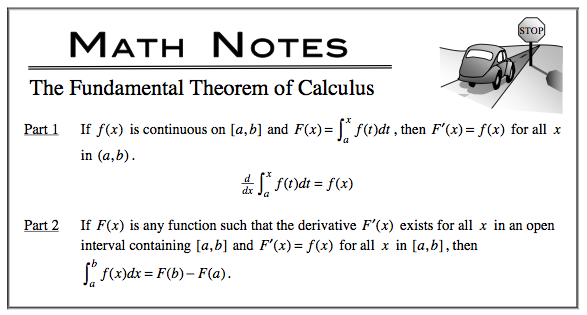### Home > CALC > Chapter 6 > Lesson 6.4.2 > Problem6-142

6-142.

Without using your calculator, evaluate the following expressions.1. $\frac { d } { d x } ( \int _ { 2 } ^ { x } \sqrt { 9 - x ^ { 3 } } d x )$1. $\frac { d } { d t } ( t ^ { - 1 } \operatorname { sin } t )$

Product Rule or Quotient Rule, depending how you write $t ^{-1}$.

1. $\int [ \frac { d } { d x } ( \frac { x + \operatorname { tan } x } { x - \operatorname { sec } x } + 5 ) ] d x$

Refer to the hint in part (a). But notice that this is an integral of a derivative, not the reverse. That means that this answer will include $+C$.

1. $\int _ { 0 } ^ { 1 } [ \frac { 1 } { \sqrt { 1 - x ^ { 2 } } } + \operatorname { sec } ^ { 2 } x ] d x$

Both parts of the sum in the integrand have antiderivatives that are trig (or inverse trig) functions.